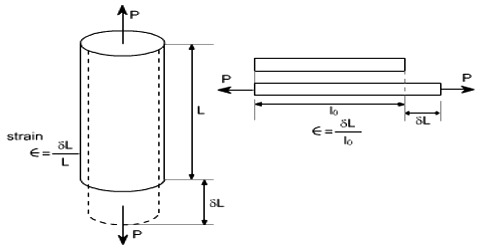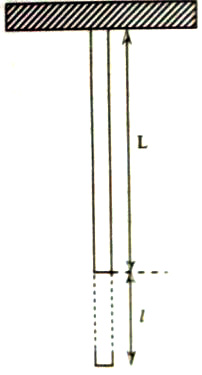Physics

# What is Longitudinal Strain?Longitudinal strain: If there is a change in the length of a body due to the applied force, then the strain is called longitudinal strain. It is the ratio of the change in length of a body to the original length of the body. It is measured by the change in length per unit length. This kind of strain is also called torsion strain.

It is defined as – change in the length to the original length of an object. It is caused due to longitudinal stress and is denoted by the Greek letter epsilon 𝜺.

Let the initial length of a body = L

The change in length due to applied force = l (Figure)

Longitudinal strain = l/LIf the length increases due to tensile stress, the corresponding strain is called tensile strain. If the length decreases due to compressive stress, the strain is called compressive strain. The longitudinal strain, e, is measured in terms of the changes in the lengths of lines.

Stress and strain are like two sides of a coin. It is important to know their behavior at microscopic scales. The longitudinal strain derived from the pressure load comprises two components: the capped-end effect strain caused by the longitudinal pressure difference in the capped end and the Poisson’s ratio effect strain due to the contributions of the hoop and radial stresses.

In Mechanics, the strain is defined as the ratio of change in dimension to the original dimension of a body when it is deformed. Each atom or molecule surrounded by neighboring atoms or molecules is bonded together by intermolecular forces and stay in equilibrium. It is a dimensionless quantity as it is a ratio between two quantities of the same dimension. When a deforming force or external force is applied, their intermolecular or inter-atomic distances vary.

When a body is under load, it will extend in the direction of the stress (longitudinal strain) and contract in the transverse or lateral direction (lateral strain), in case of longitudinal tensile stress. As a result, a restoring force will be generated to attain back the equilibrium, thereby regaining the original size and shape.